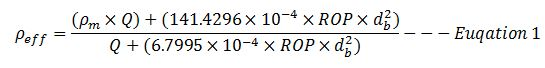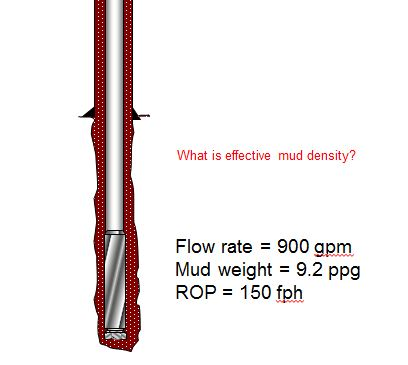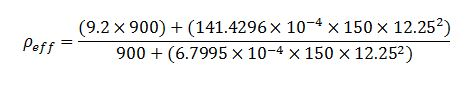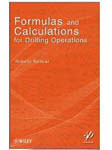# Increase In Mud Weight Due To Cutting

Cutting generated while drilling will increase drilling fluid density and it will finally affect equivalent circulating density while drilling. In this topic, we will talk about how to determine mud weight increase due to cutting.Figure 1 – Cutting Increases Mud Density

Effective mud density due to cuttings in the hole can be determined by the empirical equation below;Where;

ρeff is effective mud density in ppg.

ρm is mud density in ppg.

Q is flow rate in gpm.

ROP is rate of penetration in fph.

db is wellbore diameter or bit diameter in inch.

The effective mud density is the combination of drilling mud density and cutting density. We can write in term of equation below.

ρeff = ρm + ρc — Equation 2

Equivalent Circulating Density (ECD) consists of three components given by the equation below;

ECD = ρm + ρc + ρa — Equation 3

Where;

ρeff is effective mud density in ppg.

ρm is mud density in ppg.

ρc is cutting density in ppg.

ρa is annular pressure loss in ppg.

As you can see from the equation 1 and 3, the more the well is drilled, the more effective mud density which will increase ECD of the well.

Example: The well was drilled with 12-1/4” bit and the average ROP is 150 fph. Flow rate while drilling is 900 gpm and the rig was using 9.2 ppg mud (Figure 2). Determine the effective mud density from the information.Figure 2 – Drilling information

The parameters given are listed below;

ρm = 9.2 ppg

Q = 900 gpm

ROP = 150 pfh

db = 12.25 inch

Using the equation#1, we will get the effective mud density.ρeff = 9.39 ppg

The effective mud density is 9.39 ppg. It means that you will have 2% increase in mud weight due to cutting with these drilling parameters.

Reference book =>Formulas and Calculations for Drilling Operations

Share the joy1.al amry abdullah says: